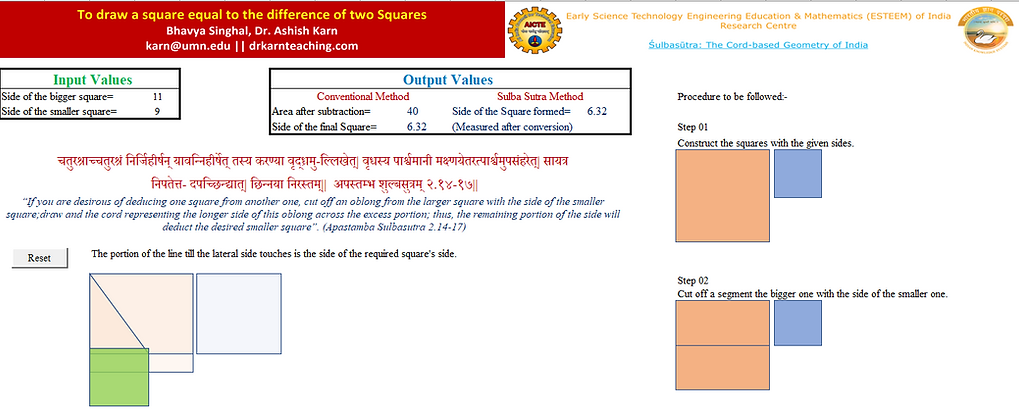top of page
##### Deducting two unequal squares

Baudhayana, Apastambha, and Katyayana give the rule for drawing a square equivalent to the difference of two unequal squares,

According to Katyayana Sulbasutra,

चतुरस्त्राच्चतुरस्त्रं निर्जिहीर्षन्यावन्निर्जिहीर्षेत्तावदुभयतोऽ पच्छिद्य शङ्कू निखाय पार्श्वमानीं कृत्वा पार्श्वमानीसम्मितामक्ष्णयां तत्रोपसंहरति,स समासेऽपच्छेदः, सा करण्येष निर्ह्वासः|| कात्यायन शुल्बसुत्रम् ३.१||

“If you wish to deduct a square from another one, cut off a rectangular portion from the larger square such that one side of that portion is equal to the side of the larger square and the other side is equal to the side of the smaller square. Draw a longer side of this portion diagonally across to the other long side. Cut off that portion where it touches the other side".

Baudhayana gives the rule as,

“To deduct a square from a square, cut off from the larger(rectangular) segment with a side of the square which is to be deducted.  Then draw a longer side of this segment diagonally across to the other long side; and where it falls (on the other side, cut off that portion. By this cut-off portion, the deduction is finished.”(Dutta, 1932)Let ABCD be the larger square and NOPQ the smaller square, Cut a rectangular portion of AEFD from the larger square such that AE= DF= the side of NOPQ. From F as the center, draw an arc with a radius ED towards AD intersecting it at G. GD will be the side of the required square GDIH.

In the right-angle triangle GDF,

GD2 = GF2 – DF2

□ GDIH = □ ABCD - □ NOPQ

Apastambha gives the rule with an illustrative example,

“The side-drawn cross becomes the diagonal (of a certain rectangle). Let it be the generator of the four-fold (of a certain square). It produces (square equal to) both the squares which are produced separately by the cut-off portion of the opposite side) and the other side (i.e., the shorter side of the rectangular segment of the larger square or the side of the other square). If the other side is one purusha, the remainder will be three square purusha. It has been stated before”.(Dutta, 1932)

Computational tool for deducting a square from another,